# [PYTHON] Kernel SVM (make_circles)

## ■ Introduction

This time, I will summarize a simple kernel SVM implementation.

[Target readers] ・ Those who want to learn simple code of kernel SVM ・ Those who do not understand the theory but want to see the implementation and give an image, etc.

## ■ Kernel SVM procedure

Proceed with the next 7 steps.

1. Preparation of module
2. Data preparation
3. Data visualization
4. Creating a model
5. Model plot
6. Output of predicted value
7. Model evaluation

## 1. Preparation of module

First, import the required modules.

``````
import numpy as np
import pandas as pd
import matplotlib.pyplot as plt
import seaborn as sns

from sklearn.datasets import make_circles
from sklearn.preprocessing import StandardScaler
from sklearn.model_selection import train_test_split
from sklearn.svm import SVC
from sklearn.metrics import roc_curve, roc_auc_score
from sklearn.metrics import accuracy_score, f1_score
from sklearn.metrics import confusion_matrix, classification_report
``````

## 2. Data preparation This time, we will use the dataset called make_circles provided by sklearn.

First get the data, standardize it, and then split it.

``````
X , y = make_circles(n_samples=100, factor = 0.5, noise = 0.05)

std = StandardScaler()
X = std.fit_transform(X)
``````

In standardization, for example, when there are 2-digit and 4-digit features (explanatory variables), the influence of the latter becomes large. The scales are aligned by adjusting so that the average is 0 and the variance is 1 for all features.

``````
X_train, X_test, y_train, y_test = train_test_split(X, y, test_size = 0.3, random_state=123)

print(X.shape)
print(y.shape)
# (100, 2)
# (100,)

print(X_train.shape)
print(y_train.shape)
print(X_test.shape)
print(y_test.shape)
# (70, 2)
# (70,)
# (30, 2)
# (30,)
``````

## 3. Data visualization

Let's look at the data plot before binary classification in the kernel SVM.

``````
fig, ax = plt.subplots()

ax.scatter(X_train[y_train == 0, 0], X_train[y_train == 0, 1], c = "red", label = 'class 0' )
ax.scatter(X_train[y_train == 1, 0], X_train[y_train == 1, 1], c = "blue", label = 'class 1')

ax.set_xlabel('X0')
ax.set_ylabel('X1')
ax.legend(loc = 'best')

plt.show()
``````

Features corresponding to class 0 (y_train == 0) (X0 is the horizontal axis, X1 is the vertical axis): Red Features corresponding to class 1 (y_train == 1) (X0 is the horizontal axis, X1 is the vertical axis): Blue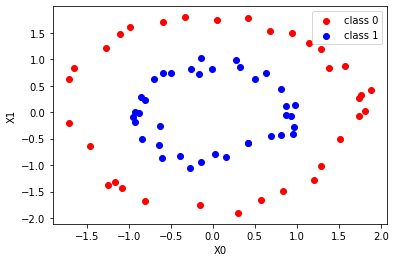The above is a bit verbose code, but it can be concise and short.

``````
plt.scatter(X_train[:, 0], X_train[:, 1], c = y_train)
plt.show()
``````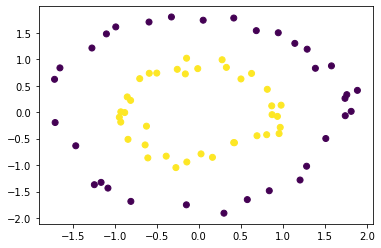## 4. Creating a model

Create an instance of the kernel SVM and train it.

``````
svc = SVC(kernel = 'rbf', C = 1e3, probability=True)
svc.fit(X_train, y_train)
``````

Since linear separation (separation by one straight line) is already impossible this time, kernel ='rbf' is set in the argument.

C is a hyperparameter that you adjust yourself while looking at the output values and plots.

## 5. Model plot

Now that you have a model of the kernel SVM, plot it and check it.

The first half is exactly the same as the scatter plot code above. After that, it's a little difficult, but you can plot other data just by pasting it as it is. (Some fine adjustment is required)

``````
fig, ax = plt.subplots()

ax.scatter(X_train[y_train == 0, 0], X_train[y_train == 0, 1], c='red', marker='o', label='class 0')
ax.scatter(X_train[y_train == 1, 0], X_train[y_train == 1, 1], c='blue', marker='x', label='class 1')

xmin = -2.0
xmax = 2.0
ymin = -2.0
ymax = 2.0

xx, yy = np.meshgrid(np.linspace(xmin, xmax, 100), np.linspace(ymin, ymax, 100))

xy = np.vstack([xx.ravel(), yy.ravel()]).T
p = svc.decision_function(xy).reshape(100, 100)
ax.contour(xx, yy, p, colors='k', levels=[-1, 0, 1], alpha=1, linestyles=['--', '-', '--'])

ax.scatter(svc.support_vectors_[:, 0], svc.support_vectors_[:, 1],
s=250, facecolors='none', edgecolors='black')

ax.set_xlabel('X0')
ax.set_ylabel('X1')

ax.legend(loc = 'best')

plt.show()
``````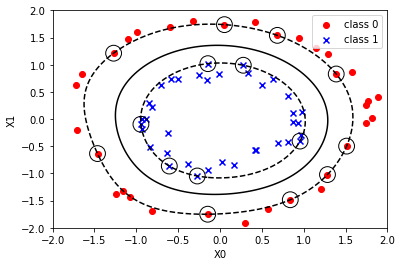## 6. Output of predicted value

With the created model, we will give the predicted value of the classification.

``````
y_proba = svc.predict_proba(X_test)[: , 1]
y_pred = svc.predict(X_test)

print(y_proba[:5])
print(y_pred[:5])
print(y_test[:5])

# [0.99998279 0.01680679 0.98267058 0.02400808 0.82879465]
# [1 0 1 0 1]
# [1 0 1 0 1]
``````

## 7. Performance evaluation Use the ROC curve to find the value of AUC.
``````
fpr, tpr, thresholds = roc_curve(y_test, y_proba)
auc_score = roc_auc_score(y_test, y_proba)
plt.plot(fpr, tpr, label='AUC = %.3f' % (auc_score))
plt.legend()
plt.title('ROC curve')
plt.xlabel('False Positive Rate')
plt.ylabel('True Positive Rate')
plt.grid(True)

print('accuracy:',accuracy_score(y_test, y_pred))
print('f1_score:',f1_score(y_test, y_pred))

# accuracy: 1.0
# f1_score: 1.0
``````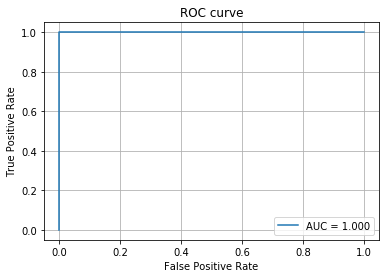``````
classes = [1, 0]
cm = confusion_matrix(y_test, y_pred, labels=classes)

cmdf = pd.DataFrame(cm, index=classes, columns=classes)

sns.heatmap(cmdf, annot=True)
print(classification_report(y_test, y_pred))

'''
precision    recall  f1-score   support

0       1.00      1.00      1.00        17
1       1.00      1.00      1.00        13

accuracy                           1.00        30
macro avg       1.00      1.00      1.00        30
weighted avg       1.00      1.00      1.00        30

'''
``````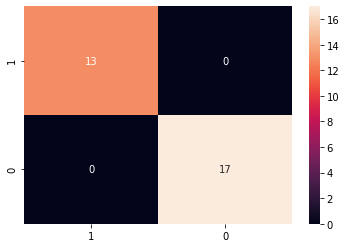## ■ Finally

Based on the steps 1 to 7 above, we were able to create a kernel SVM model and evaluate its performance.

We hope that it will be of some help to beginners.

## ■ References

Recommended Posts